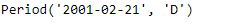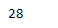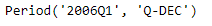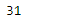# Python | Pandas Period.daysinmonth

• Last Updated : 06 Jan, 2019

Python is a great language for doing data analysis, primarily because of the fantastic ecosystem of data-centric python packages. Pandas is one of those packages and makes importing and analyzing data much easier.

Pandas` Period.daysinmonth` attribute returns the number of days in the month present in the given Period object.

Syntax : Period.daysinmonth

Parameters : None

Return : number of days

Example #1: Use `Period.daysinmonth` attribute to find the number of days present in the month in the given Period object.

 `# importing pandas as pd``import` `pandas as pd`` ` `# Create the Period object``prd ``=` `pd.Period(freq ``=``'D'``, year ``=` `2001``, month ``=` `2``, day ``=` `21``)`` ` `# Print the Period object``print``(prd)`

Output :Now we will use the `Period.daysinmonth` attribute to find the number of days present in the month represented in the given period object.

 `# return the number of days``prd.daysinmonth`

Output :As we can see in the output, the `Period.daysinmonth `attribute has returned 28 indicating that the month represented in the given period object has 28 days.

Example #2: Use `Period.daysinmonth` attribute to find the number of days present in the month in the given Period object.

 `# importing pandas as pd``import` `pandas as pd`` ` `# Create the Period object``prd ``=` `pd.Period(freq ``=``'Q'``, year ``=` `2006``, quarter ``=` `1``)`` ` `# Print the object``print``(prd)`

Output :Now we will use the `Period.daysinmonth` attribute to find the number of days present in the month represented in the given period object.

 `# return the number of days``prd.daysinmonth`

Output :As we can see in the output, the `Period.daysinmonth `attribute has returned 31 indicating that the month represented in the given period object has 31 days.

My Personal Notes arrow_drop_up